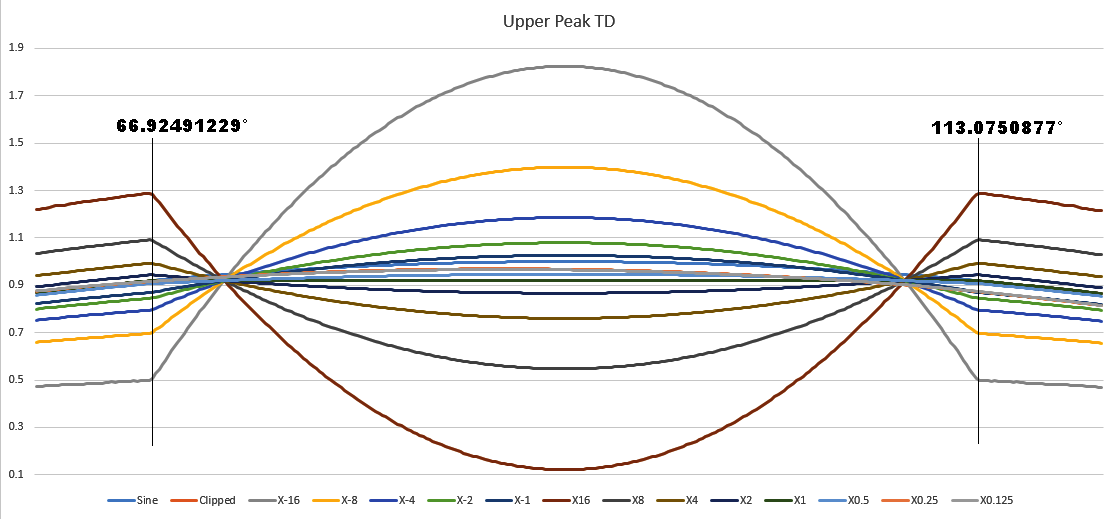Distorted cosine wave with noise

# More Fun With Inverse FFTs

Published on February 3, 2020

I was playing around with a symmetrically clipped sine wave and accidentally proved Bullard Laws of Harmonics #1, #2 and #4. Here is what I did.

As I said, I symmetrically clipped a sine wave at the usual place, 4 percent clipped off each side of the transfer function. Now that doesn't mean that 4 percent of the wave is clipped, that depends on the area of the sine wave near the peak, but to put it simply, I clipped the 1V peak sine wave at 0.92 volts and I clipped the negative peak at -0.92 volts. Then, I did an FFT on it and took the spectrum and multiplied each and every harmonic by a variable ranging from 16, 8, 4, 2, 1, 0.5, 0.25, 0.125 and -16, -8, -4, -2, -1. In frequency domain it looks like this:Did you notice that each value is exactly 6dB from the one below it? That's the factor of 2 from the progression I mentioned above (16, 8, 4, 2, 1, 0.5, 0.25, 0.125).

Now, the important thing to note here is that the positive and negative values appear identical, that is, the spectral bins for -16 looks identical to +16. So how does -16 differ from +16 if not in Frequency Domain? The difference is that in the Excel code I look at the multiplier and if it's positive I take the square root of the multiplier and use that as a multiplier in the IMPRODUCT() function to boost (or attenuate) the amplitudes of the spectral bins in complex form. However, if the multiplier is negative, I take the square root of the absolute value of the multiplier, then assign the IM side to be negative, which changes the phase of the harmonic. So if it's 90 degrees, it becomes 270 degrees, if it's 0 degrees it becomes 180 degrees, if it's 270 degrees it becomes 90 degrees and if it's 180 degrees it becomes 0 degrees, (remember, only 4 values for a sine wave according to Bullard Laws of Harmonics #5) which changes the time domain quite a bit. So we do the inverse FFT and take a look at the results.When the phase is left alone, the flat peak is driven down, down down, which might seem counter-intuitive, but it's not. When you clip the peak of a wave, the phases of the harmonics are negative to counter the bump at the top (or bottom) of the peak. So enhancing the amplitude of the harmonics will cause the flat peak to become even more negative (on the positive side) or positive (on the negative side). But, if I invert the phase, the amplitude of the peak gets boosted, so no longer is it flat, but it takes the opposite trajectory, so the positive peak goes very positive and the negative side goes very negative. This behavior proves Bullard Laws of Harmonics #4.

Now, you might wonder why the body of the wave differs from the default clipped wave. It appears that the larger the negative peak, the bigger the body of the wave, and the taller the positive peak, the smaller the body of the wave is. Why would this be? Because I have a secret I haven't admitted to yet: I held the fundamental bin amplitude constant while changing all the harmonic amplitudes. What that means is that, according to Bullard Laws of Harmonics #1, that the bin amplitude controls the area of the wave and vice versa. If I hold the fundamental amplitude rock solid, then the area of the wave with a frequency of F (whatever that frequency might be) must remain the same. So when the harmonics make the peak plunge towards zero, in order to keep the area the same, the amplitude of the body of the wave must be increased. When the harmonics make the peak get taller, to keep the area the same, the profile of the wave must be reduced, but in a sinusoidal manner to avoid distorting the wave. It's pretty obvious when you think about it.

Now, we've proved Bullard Laws of Harmonics #1 and #4, but what about Law #2 which says: The Harmonic Signature is the result of the angle where the sinusoid impacts the distortion? To show you, let's take a closeup look at the positive peak of the wave:Notice that all signals start and end their distortion at exactly the same phase angle in the sine wave. At the front side it's 66.92491229° and on the back side it's 113.9750877°. That is controlled by the harmonic signature, as we saw in the frequency domain plot above. If you modify the amplitudes of individual frequencies, the angle will change, but because I multiply every harmonic by the same value, the harmonic signature remains the same, thus the angle remains the same for each time domain wave. So once again, I've proven Law #2, which is a law that was never discovered by anybody other than me.

The only thing I still need to mention is that I held the DC bin amplitude and phase the same from experiment to experiment, otherwise if you accidentally (I did at first) multiply the DC bin by the multiplier, things don't line up very well.

Just another example of the perfection of the Bullard Laws of Harmonics, deduced by me and me alone. Not one other person on the planet ever figured this stuff out. To them, harmonics are caused by fairy farts or other inexplicable phenomena. Just don't listen to anyone else, professor, engineer, scientist or anyone else, they are all morons.